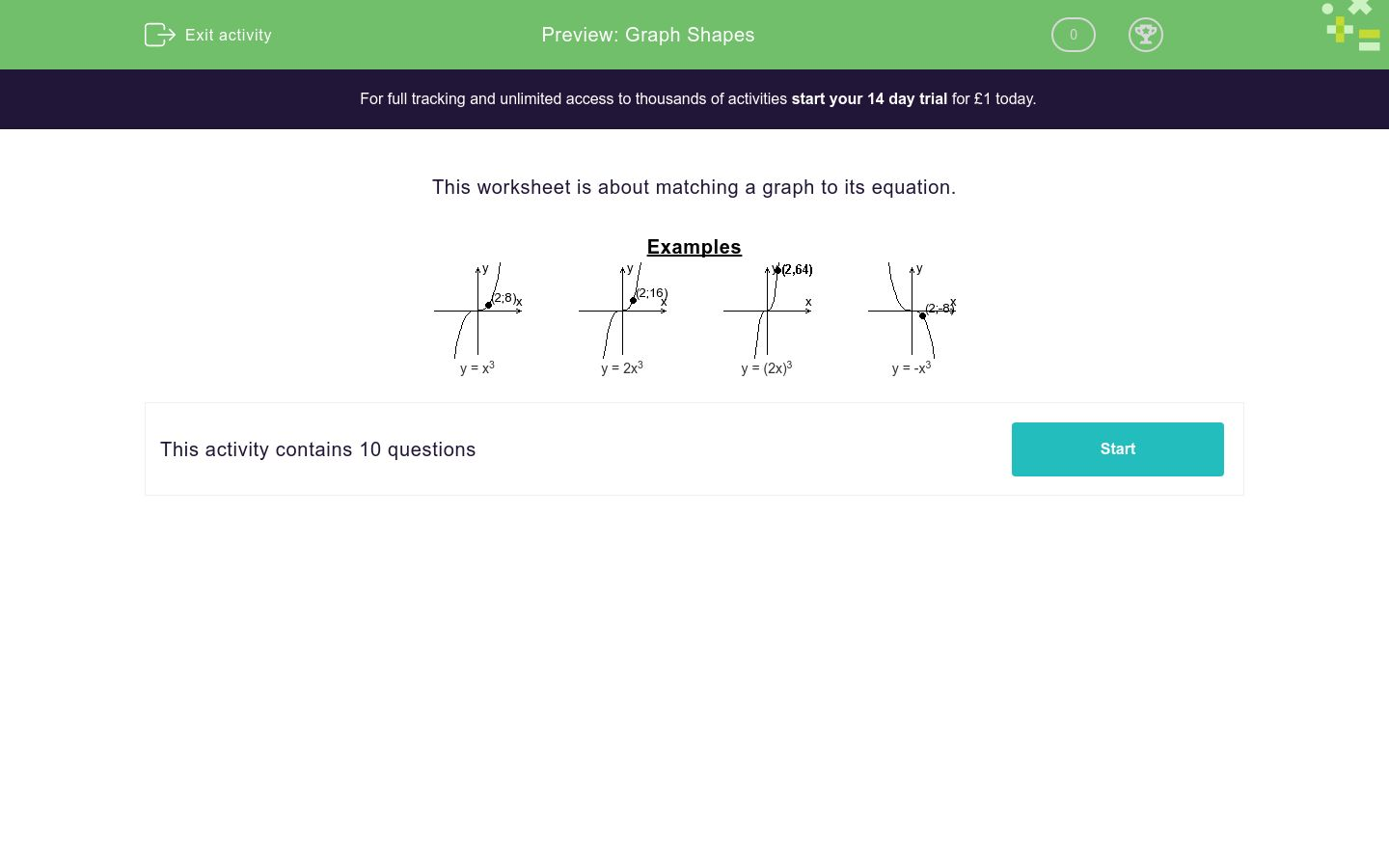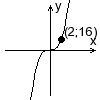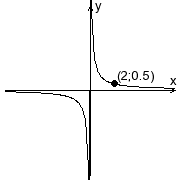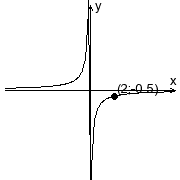# Graph Shapes

In this worksheet, students select the correct graph to match the given function.Key stage:  KS 4

Curriculum topic:  Algebra

Difficulty level:### QUESTION 1 of 10

This worksheet is about matching a graph to its equation.

Examplesy = x3y = 2x3y = (2x)3y = -x3

Which graph represents the following function?

 y = 1 xABC
A

B

C

Which graph represents the following function?

 y = - 1 xABC
A

B

C

Which graph represents the following function?

 y = 2 xABC
A

B

C

Which graph represents the following function?

 y = 3 xABC
A

B

C

Which graph represents the following function?

 y = 1 2xABC
A

B

C

Which graph represents the following function?

 y = 1 3xABC
A

B

C

Which graph represents the following function?

y = -x2ABC
A

B

C

Which graph represents the following function?

y = 2x2ABC
A

B

C

Which graph represents the following function?

y = 3x2ABC
A

B

C

Which graph represents the following function?

y = -2x2ABC
A

B

C

• Question 1

Which graph represents the following function?

 y = 1 xABC
B
EDDIE SAYS
0.5 = 1 / 2
• Question 2

Which graph represents the following function?

 y = - 1 xABC
C
EDDIE SAYS
-0.5 = -1 / 2
• Question 3

Which graph represents the following function?

 y = 2 xABC
A
EDDIE SAYS
1 = 2 / 2
• Question 4

Which graph represents the following function?

 y = 3 xABC
A
EDDIE SAYS
1.5 = 3 / 2
• Question 5

Which graph represents the following function?

 y = 1 2xABC
B
EDDIE SAYS
0.25 = 1 / (2 × 2)
• Question 6

Which graph represents the following function?

 y = 1 3xABC
C
EDDIE SAYS
0.17 = 1 / (3 × 2)
• Question 7

Which graph represents the following function?

y = -x2ABC
A
EDDIE SAYS
-4 = - (2)²
• Question 8

Which graph represents the following function?

y = 2x2ABC
B
EDDIE SAYS
8 = 2 × (2)²
• Question 9

Which graph represents the following function?

y = 3x2ABC
B
EDDIE SAYS
12 = 3 × (2)²
• Question 10

Which graph represents the following function?

y = -2x2ABC
C
EDDIE SAYS
-8 = -2 × (2)²
---- OR ----

Sign up for a £1 trial so you can track and measure your child's progress on this activity.

### What is EdPlace?

We're your National Curriculum aligned online education content provider helping each child succeed in English, maths and science from year 1 to GCSE. With an EdPlace account you’ll be able to track and measure progress, helping each child achieve their best. We build confidence and attainment by personalising each child’s learning at a level that suits them.